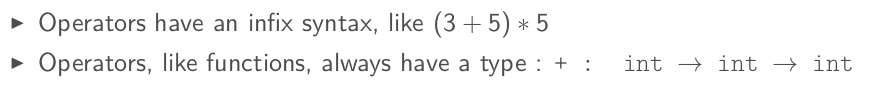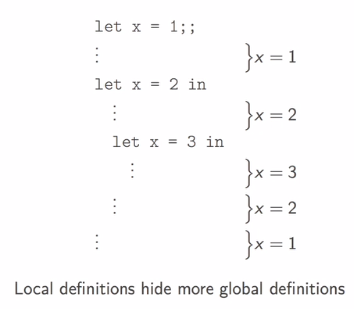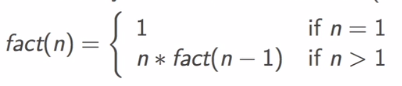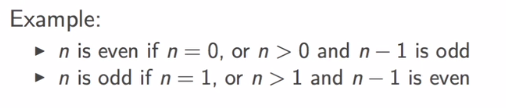# 1. BASIC DATA TYPES: int, bool

Rich type system and polymorphism in ocaml. Types are infered not declared.

Basic types: int, bool, float, string, char, ...

### int

value: $$-2^{62}$$ ~ $$2^{62}-1$$ on 64-bit machines.
ops: +, -, *, /, mod (reminder: / is integer division)

# 3+2*4-1;;
- : int = 10
# 5/2;;
- : int = 2
# -5 mod 3;;
- : int = -2


### bool

values: true, false ops: &&,||, not comparison ops: <, >, =, <=, =>, <>
note 1: comparisons must have the same type of operands) note 2: equal test is = , = is NOT assigment. == exists but is for something else...

# true && (1<7);;
- : bool = true
# 1.0 < 7;;
Error: This expression has type int but an expression was expected of type
float
# 5 > "hello";;
Error: This expression has type string but an expression was expected of type
int
# 3=3;;
- : bool = true
# 3==3;;
- : bool = true
# 3<3.0;;
Error: This expression has type float but an expression was expected of type
int
# 3.0 < 3.0;;
- : bool = false


# 2. MORE DATA TYPES: float, char, string

### float

value: must written with a dot (5.0, 5.), or exponential (5e3, 6e-9) ops: also have a dot in the end +., -., *., /. functions: sqrt, sin, cos, ceil, floor, ...

# 3.0 +. 1e10;;
- : float = 10000000003.
# 2 + 3.0;;
Error: This expression has type float but an expression was expected of type
int
# 3. +. 2.0;;
- : float = 5.


All basic types are disjoint: no value belongs to 2 different basic types, no implicit conversion.

conversion functions in both directions:

• float_of_int: int -> float
• int_of_float: float -> int
# float_of_int(2);;
- : float = 2.
# int_of_float(2.4);;
- : int = 2
# float_of_int 2;;
- : float = 2.
# int_of_float 2.8;;
- : int = 2


Parentheses only necessary to indicate structure.

### char

values: 256 chars (0~255), can be written as 'a' or '\087' conversion functions (char ↔ int):

• Char.chr: int ->char
• Char.code: char -> int
# Char.code 'A';;
- : int = 65
# Char.code '\122';;
- : int = 122
# Char.chr 233;;
- : char = '\233'
# Char.chr 65;;
- : char = 'A'
# Char.chr (Char.code 'B');;
- : char = 'B'


### string

values: written between "" ops: ^ for string concatenation functions: String.length, int_of_string, float_of_string, String.get(s,i) ...

# "abc" ^ "def";;
- : string = "abcdef"
# String.length "1234";;
- : int = 4
# int_of_string "123";;
- : int = 123
# int_of_string "123a";;
Exception: Failure "int_of_string".
# string_of_int 123;;
- : string = "123"
# String.get "abc" 1;;
- : char = 'b'


# 3. EXPRESSIONS: if-then-else, func-applications and operators

expressions are used to compute values.

### conditional expr

if... then... else... ⇒ this gives an expression, not an instruction.

An expression always have a type, type of the if-then-else expression is the type of the expressions in then and else, which must be the same.

If the else is missing → there is a default value, to be discussed later

# if 1<2 then 8 else 9;;
- : int = 8
# if 6=8 then 1 else 8;;
- : int = 8
# if 6=8 then 1 else 8.;;
Error: This expression has type float but an expression was expected of type
int
# if(if 1=1 then 2=2 else 3>2) then 2<3 else 3<2;;
- : bool = true


### function applicationagain, () are not needed unless indicate structure, and parentheses are NOT function applications...

# String.get;;
- : string -> int -> char = <fun>
# String.get "abcde" 2;;
- : char = 'c'
# String.get ("Hello"^"world") (3+2);;
- : char = 'w'
# String.get (string_of_int 65) (int_of_string "0");;
- : char = '6'
# String.get("hello", 2);;
Error: This expression has type 'a * 'b
but an expression was expected of type string


### Polymorphic operatorsSome ops have polymorphic(generic) types, like >: 'a → 'a → bool Here the 'a is a type variable, can be instantiated by any type.

# 12 > 23.0;;
Error: This expression has type float but an expression was expected of type
int
# 'a' > 'b';;
- : bool = false
# "hello" > "world";;
- : bool = false


# 4. DEFINITIONS

definitions are used to give names to values, in ocaml there are global defs and local defs.

### global definition

effective for rest of session syntax: let name = expr once set, the value of the identifier never changes.

# let x = 2+3;;
val x : int = 5
# let y = 2*x;;
val y : int = 10
# let x = 3;; # **the old definition of x will be shadowed**
val x : int = 3
# x = x+1;;
- : bool = false


### local definition

naming within a delimited scope syntax: let name = expr1 in expr2 the scope of the identifier is expr2, the result's value is also expr2's value. So the identifier should appear in expr2.

# let x = 9 in 2*x;;
- : int = 18
# x;; # x is not in global scope
Error: Unbound value x
# let y = x+1 in y/3;;
Error: Unbound value x
# let x = 17;; # now x is global
val x : int = 17
# let y = x+1 in y/3;;
- : int = 6


local defs can be nested:

# let x = 4 in
let y = x+1 in
let x = 2*y in x;;
Error: Syntax error
# let x = 4 in
let y = x+1 in
let x=2*y in x;;
- : int = 10
# let x = 4 in
(let x=17 in x+1) + x;;
- : int = 22### Simultaneous Definitions

can assign 2 identifiers in the same line:

    # let x1=2 and x2=3-3;;
val x1 : int = 2
val x2 : int = 0


Note: expr is evaluated w.r.t. the value bindings before the let.

# let x=2 in
let y=x+1 in (*y=2+1*)
x*y;;
- : int = 6
# let x=1;;
val x : int = 1
# let x=2 and y=x+1 in (*y=1+1 because the binding for x before the let is 1!*)
x*y;;
- : int = 4


# 5. FUNCTIONS

### func definition

global function (with 1 argument) def : let f x = expr

local def: let f x = expr1 in expr2

# let f x = x+1;;
val f : int -> int = <fun>
# f 17;;
- : int = 18
# let g y = 2*y
in g 42;;
- : int = 84
# f f 1;; (* interpreted as apply 2 argments to f, need parentheses here*)
Error: This function has type int -> int
It is applied to too many arguments; maybe you forgot a ;'.
# f (f 1);;
- : int = 3


### lexical scoping

def. lexical scoping identifiers(names) used in function body refers to the names that are visible at the moment of function definition.

def. dynamic scoping names refer to names that are visible at the moment of function invocation.

# let f x = x+1 in
let g y = f (f y) in (*here f is the f defined above, which is visible at the moment of g's declaration*)
let f x = 2*2 in (*this f is visible after g's declaration, it is visible when g is invocated*)
g 5;; (*g uses the first f*)
Warning 26: unused variable f.
- : int = 7
(*the same is true for global scoping*)
# let f x = x+1;;
val f : int -> int = <fun>
# let g y = f (f y);;
val g : int -> int = <fun>
# let f x = 2*x;;
val f : int -> int = <fun>
# g 5;;
- : int = 7


### identifiers are NOT variables

A name(identifier) can be hidden (the value is NOT changed) by a new definition of the same name.

The hidden identifier can be accessed with static(lexical) binding:

# let a = 1;;
val a : int = 1
# let f x = x+a;;
val f : int -> int = <fun>
# f 2 ;;
- : int = 3
# let a = 73;;
val a : int = 73
# f 2;; (*f still uses a's value as 2, the old value of a is NOT changed, it's hidden --closure? *)
- : int = 3


# 6. RECURSION

rec functions are natural on recursively defined data structures.

example: fact(n)### recursive definition

using the f in let's expr will cause pb as f refers to the previous value of f. ⇒ use key word rec.

# let fact n = if n<=1 then 1 else n*fact(n-1);;
Error: Unbound value fact
# let rec fact n = if n<=1 then 1 else n*fact(n-1);;
val fact : int -> int = <fun>
# fact 10;;
- : int = 3628800


### mutually recursive function

ex. 2 funcs calling each other recursivelyuse simultaneous defs (let...and...):

# let rec even x = if x=0 then true else odd(x-1)
and odd x = if x=0 then false else even(x-1);;
val even : int -> bool = <fun>
val odd : int -> bool = <fun>
# even 17;;
- : bool = false
# odd 10;;
- : bool = false


# exercices

let multiple_of n d =
(n mod d) == 0;;

let integer_square_root n =
let sqr = sqrt (float_of_int n) in
int_of_float sqr;

let rec gcd n m =
let big = max n m and small = min n m in
let r = big mod small in
if r=0 then small
else gcd r small;;

let rec multiple_upto n r =
if r<2 then false
else if multiple_of n r then true
else multiple_upto n (r-1);;

let is_prime n =
if n<=1 then false
else not (multiple_upto n (n-1));;
`